## Chapter 5: Exercises

1. Doppler effect:
1. Use your own words to describe Doppler effect.
2. Give the formula of Doppler effect.
2. Beat:
1. Use your own words to describe the phenomenon of beat.
2. Use a math formula to explain why beat occurs.
3. If two sinusoidal audio sources of frequencies 440-Hz and 446-Hz, respectively, are played at the same time, what is the beat frequency you will hear?
3. Parameters for recordings:
1. What are the three most important recording parameters (excluding recording duration) you need to specify before starting recording your speech?
2. What are the meanings of these parameters?
4. Basic speech features:
1. What are the three basic perceptible acoustic features for speech signals?
2. How do you control these features during your pronunciation?
5. (*) Audio data size: Compute the audio data size of the following specs:
1. 1 minute of recording with 16000Hz sample rate, 16 bits of resolution, and single channel
2. 3 minutes of a CD audio track with 44.1KHz sample rate, 16 bits of resolution, and 2 channels
6. (*) Frame size, overlap, and frame rate: Suppose we are dealing with an audio signal of sample rate 16000Hz, with a frame size of 512.
1. If the overlap is 112, what is the frame rate (no. of frames per second)?
2. If the frame rate is 100 frames/sec, what is the overlap (in terms of sample points)?
7. (*) Label silence, unvoiced, and voiced: Please label "silence" (no sound at all), "unvoiced" (sound without pitch) and "voiced" (sound with pitch) parts of the following waveform of my pronunciation of "such a nice place".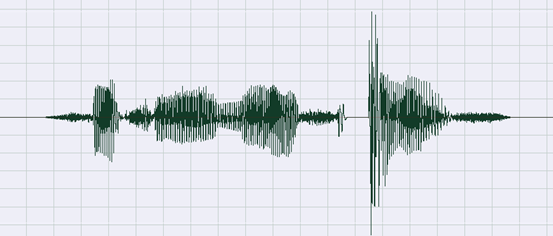1. Suppose a frame of size $n$ is represented by $\mathbf{s}=[s_1, s_2, ..., s_n]$. Give two commonly used formulas for computing the volume of this frame.
2. What are the 3 major factors that influence the perceived volume?
9. (*) Zero justification via constant: Prove the following two identities:
1. $\arg \min_x \sum_{i=1}^n (s_i-x)^2 = mean([s_1, s_2, \dots, s_n])$

Hint
Proof by setting the derivation of the objective function with respect to x to zero.

2. $\arg \min_x \sum_{i=1}^n |s_i-x| = median([s_1, s_2, \dots, s_n])$.

Hint
Proof by induction.

10. (*) Zero justification via polynomial fitting: During speech recording, it is likely that the recorded speech signals will oscillate around a non-zero time-varying value due to several reasons, including static effects, breath over the mic, and 50Hz AC voltage signals. To avoid such drifting within a frame, a simple method is to identify the time-varying zero curve via polynomial fitting, and remove the drifting by subtracking the curve from the original frame. Here is an example of such a situation when the order of the fitting polynomial is 3: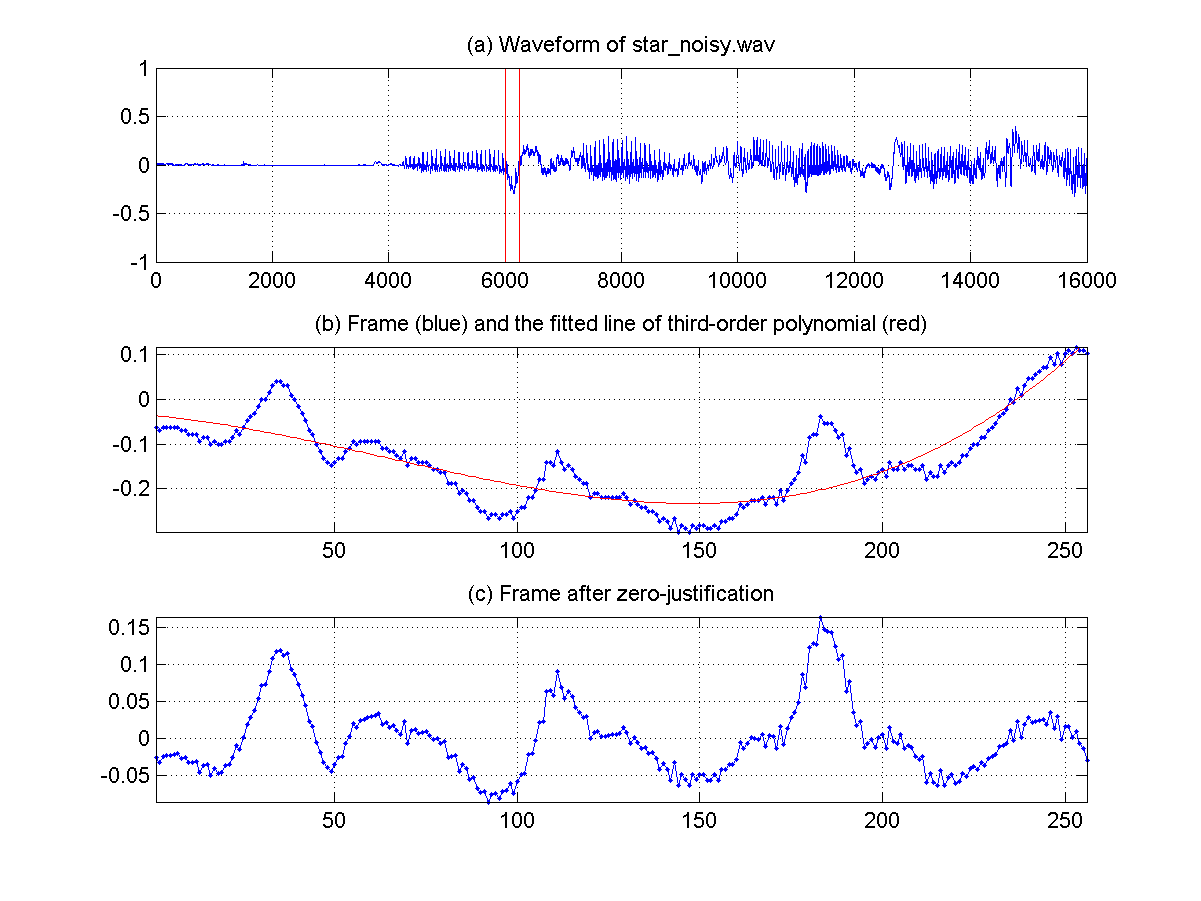Write a function frameZeroJustify.m to perform such zero-justification for a given frame matrix, with the following usage: frameMat2=frameZeroJustify(frameMat, polyOrder); where "frameMat2" is the output frame matrix, "frameMat" is the input frame matrix, and "polyOrder" is the order of the fitting polynomial. (Note that in frameMat and frameMat2, each column is a frame of audio signals.)

Here is a test exmaple using 3-order polynomial: frameMat.mat and frameMat2.mat

Hint
• The process of "zero justification" is perform on each frame independently.
• To avoid numerical error, you'd better perform z-normalization on the x-axis data for polynomial fitting first.
• Related MATLAB commands: polyfit, polyval, mean, std, etc.

11. (*) What is 440Hz: The formula for computing the pitch in semitone from the pitch in Hertz is $semtone=69+12 log_2 \left(\frac{hertz}{440}\right)$. Please explain the meaning of 440.
12. Change in sample rate during playback: If an audio clip has a sample rate of $f_s$, but we change it to $k*f_s$ during playback.
1. What will be the new duration in terms of the old duration $d$?
2. What will be the new fundamental frequency in Hz in terms of the old fundamental frequency $f_{Hz}$?
3. What will be the new fundamental frequency in Semitone in terms of the old fundamental frequency $f_{Semitone}$?
13. Pitch difference due to change in playback sample rate: If I record my voice at sample rate $f_s$ but do a playback at sample rate $\sqrt{2}f_s$, what will be the pitch difference from the original utterance?
14. Pitch curves for each tone: Plot the basic pitch curve for each of the 4 tones in Chinese Mandarin.
15. Tone Sandhi: Please label the tones of the following sentence when pronounced by a native speaker of Mandarin Chinese):
老李買好酒請馬小姐買幾百把小雨傘
(If you are a foreign student, please indicate so and you can skip this one.)
16. Frame size and peak picking of ACF: Suppose we are dealing with an audio signal of sample rate 16000Hz, and the range of human fundamental frequency (in Hz) is [100, 1000].
1. What is the reasonable minimum frame size?
2. What is the reasonable index range to find the peak in ACF in order to compute the pitch?
17. (*) Pitch computation: The waveform of a frame of my speech is shown next. Please explain how you compute the fundamental frequency (in terms of Hz) if the sample rate is 8kHz. (Note that you should try to select as many fundamental periods as possible.)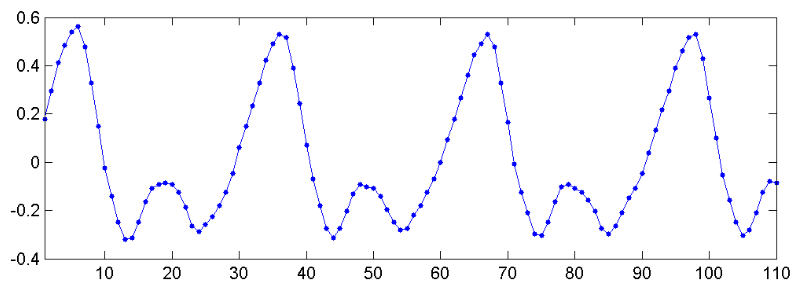18. (*) Pitch trends of the 4 tones in Mandarin: Please draw the pitch trend of the 4 tones in Mandarin Chinese.
19. (*) Conversions between Hertz and semitone: What is the formula to convert pitch in Hertz to pitch in semitone?
20. (*) Frequency-to-pitch conversion: Write an m-file function that convert a given frequency in Hz into the pitch in semitones. The format is

pitch = 69 + 12*log2(freq/440)

21. (*) Pitch-to-frequency conversion: Write an m-file function that converts a given pitch in semitones into the frequency in Hz. The format is

freq = 440*2^((pitch-69)/12)

22. (**) Visual inspection of pitch: Please follow the example of pitch determination by visual inspection to identify the pitch (in terms of semitones with two digits of precision after the decimal point) of the following recordings. Be sure to plot your result for each recording, just like what we did in the example.
1. Write a script for the clip of another sound fork, tuningFork02.wav.
2. Write a script for the first vowel part of the utterance "sunday", sunday.wav
3. Write a script to determine the highest pitch of the artist Vitas.

Hint
Please search "Vitas" at "www.youtube.com" to obtain his singing clips. You should select a high-pitch segment with as little background music as possible for your analysis.

4. (For Chinese) Write a script to find the lowest and highest pitch of 林志炫 in this clip.
5. Try your best to record two clips, one with your highest pitch possible, the other with your lowest pitch possible. The recordings should be at least 3 seconds, and make sure your pronunciation is stable such that the fundamental periods can be clearly observed in your plot. What is your pitch range? What are the corresponding keys in a piano keyboard? Be sure to submit your recordings and your programs, and show your plots to TA. Some reference results:
• 2014: Lowest (64.257 Hertz or 35.6931 semitone) and highest (1438.04 Hz or 89.5024 semitone).
23. (**) Frame-to-volume computation: Write an m-file function which can compute the volume of a given frame. The format is

volume = frame2volume(frame, method);

where "frame" is the input frame and "method" is the method for volume computation (1 for abs. sum; 2 for log square sum), and "volume" is the output volume.
24. (**) Wave-to-volume computation: Write an m-file function which can compute the volume of a given wave signals. The format is

volume = wave2volume(wave, frameSize, overlap, method);

where "wave" is the input wave signals, "frameSize" is the frame size, "overlap" is the overlap, "method" is the method for computing the volume (1 for abs. sum; 2 for log square sum), and "volume" is the output volume vector.
25. (*) Volume vs. timbre: Write an m-file script that can record your utterance of "ㄚ、ㄧ、ㄨ、ㄝ、ㄛ" (example) with a sample rate of 16 KHz and a bit resolution of 16 bits. When doing the recording, please try your best to keep a constant perceived volume for all these five vowels. Then your program should plot the wave signals together with two volume vectors (with frame size = 512, hop size = 160) computed via the two methods mentioned in this chapter. The obtained plots should be similar to the following image: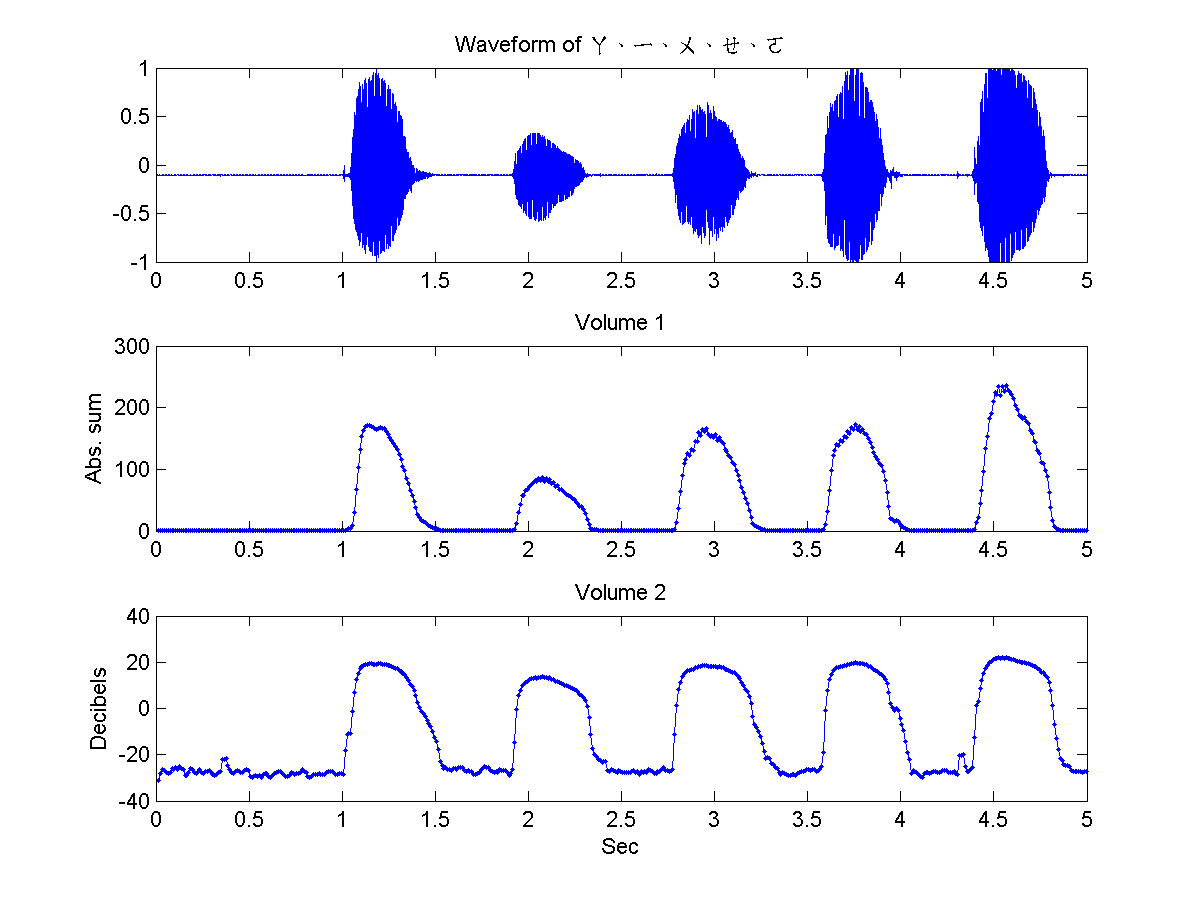From your plot, can you deduct the relationship between the volume and the shapes of your mouth? Try other vowels to see if your argument still holds.
26. (**) Use sinusoids to synthesize waveform of given volume and pitch: In the following three sub-exercises, you are going to use the sine function to synthesize audio signals with given volume and pitch.
1. Write an m-file script that uses a sinusoid of 0.8 amplitude to generate a 0.5-second mono signals with a pitch of 69 semitones, sample rate of 16 KHz. Your program should plot the waveform and play the signals. (Here is an example). The plot should look like this: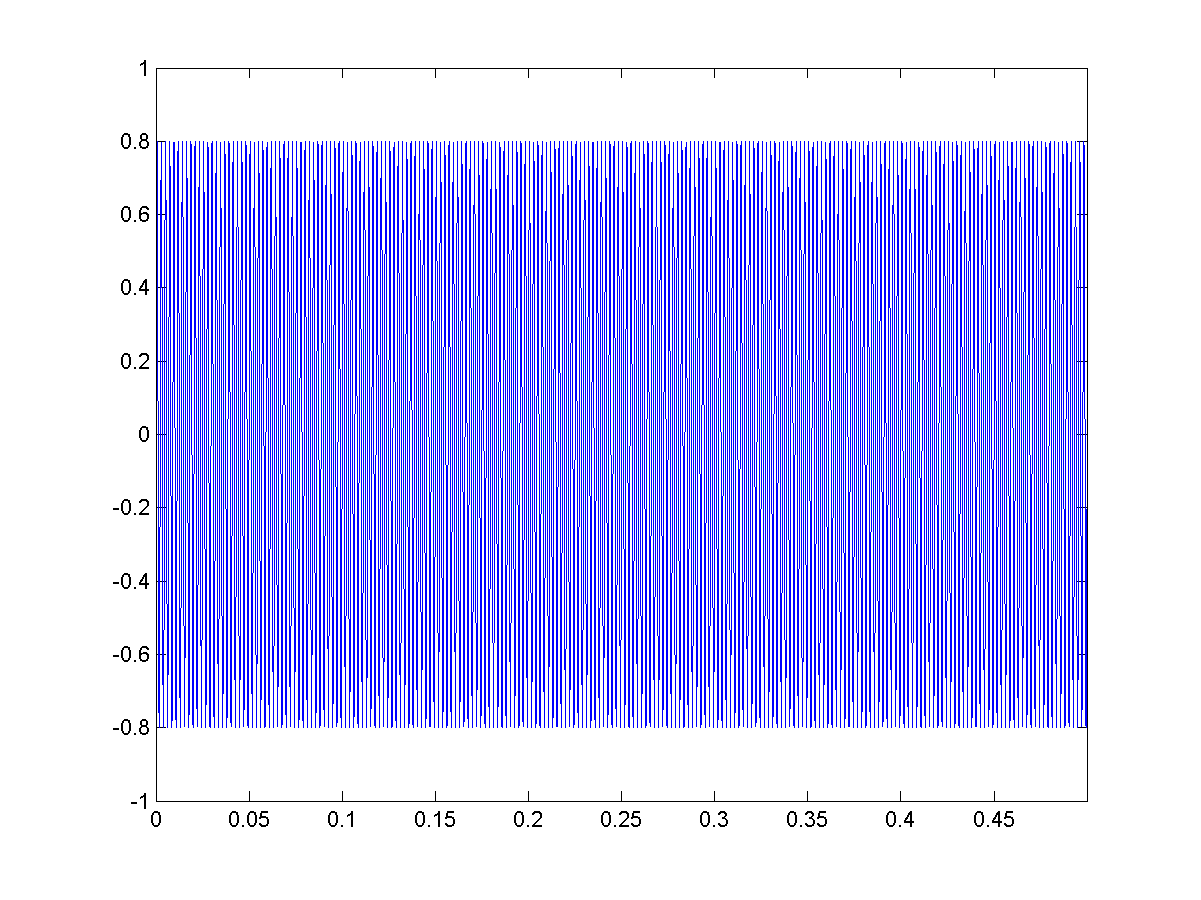Does the pitch sound the same as the recording of a tuning fork tuningFork01.wav? (Hint: a sinusoid with frequency f can be expressed as y = sin(2*pi*f*t).)
2. Write an m-file script that uses the sinusoid to generate a mono wave signal of duration of 2 seconds, pitch of 60 semitones, sample rate of 16 KHz, bit resolution of 16 bits. Moreover, the waveform should be oscillate between 0.6 and 1.0 with a frequency of 5 Hz. Your program should plot the waveform and play the signal. (Here is an example). The plot should look like this: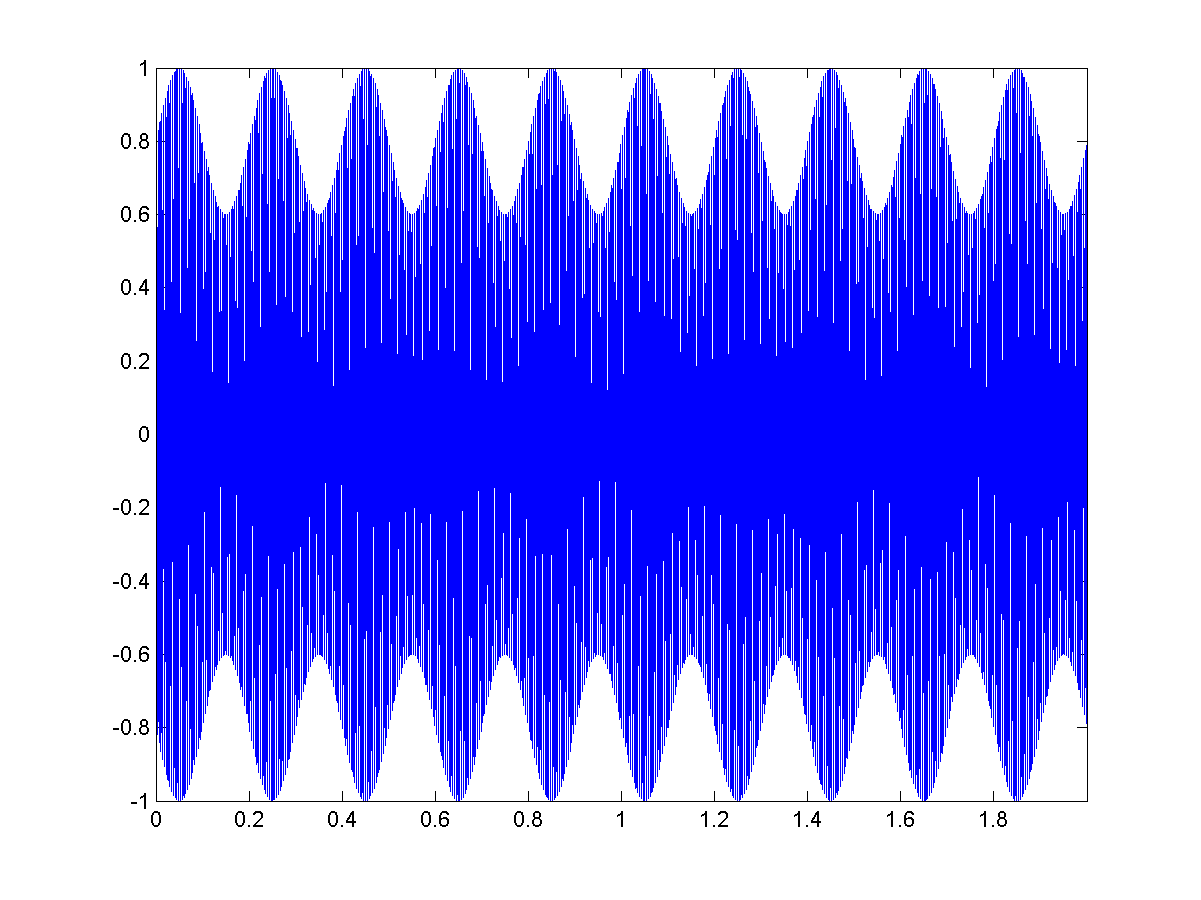3. Write an m-file script to repeat the previous sub-problem, but the intensity of the waveform should decrease by following an exponential function exp(-t). Here is an example. Your plot should look like this: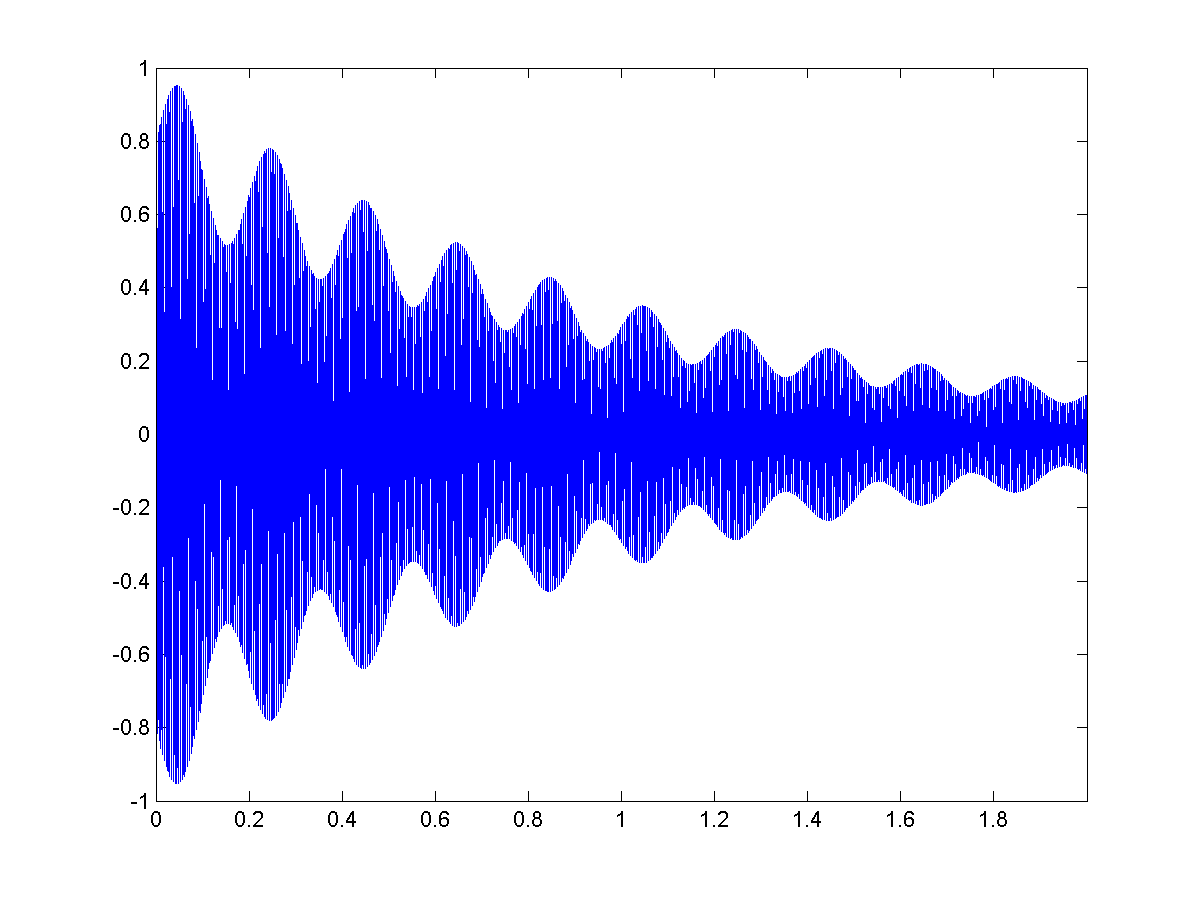27. (***) Impact of frame sizes on volume: First of all, you need to record your utterance of "beautiful sundays" and save it to a wave file of 16 KHz, 16 bits, mono. My example is here. But please use your own recording instead of mine.
1. Write an m-file script that reads the wave file and plot the volumes (as the absolute sum of a frame) with respect to frame sizes of 100, 200, 300, 400 and 500, and overlap of 0. Please use the same time axis for the first plot of the waveform, and the second plots of the 5 volume curves. You plot should look like this: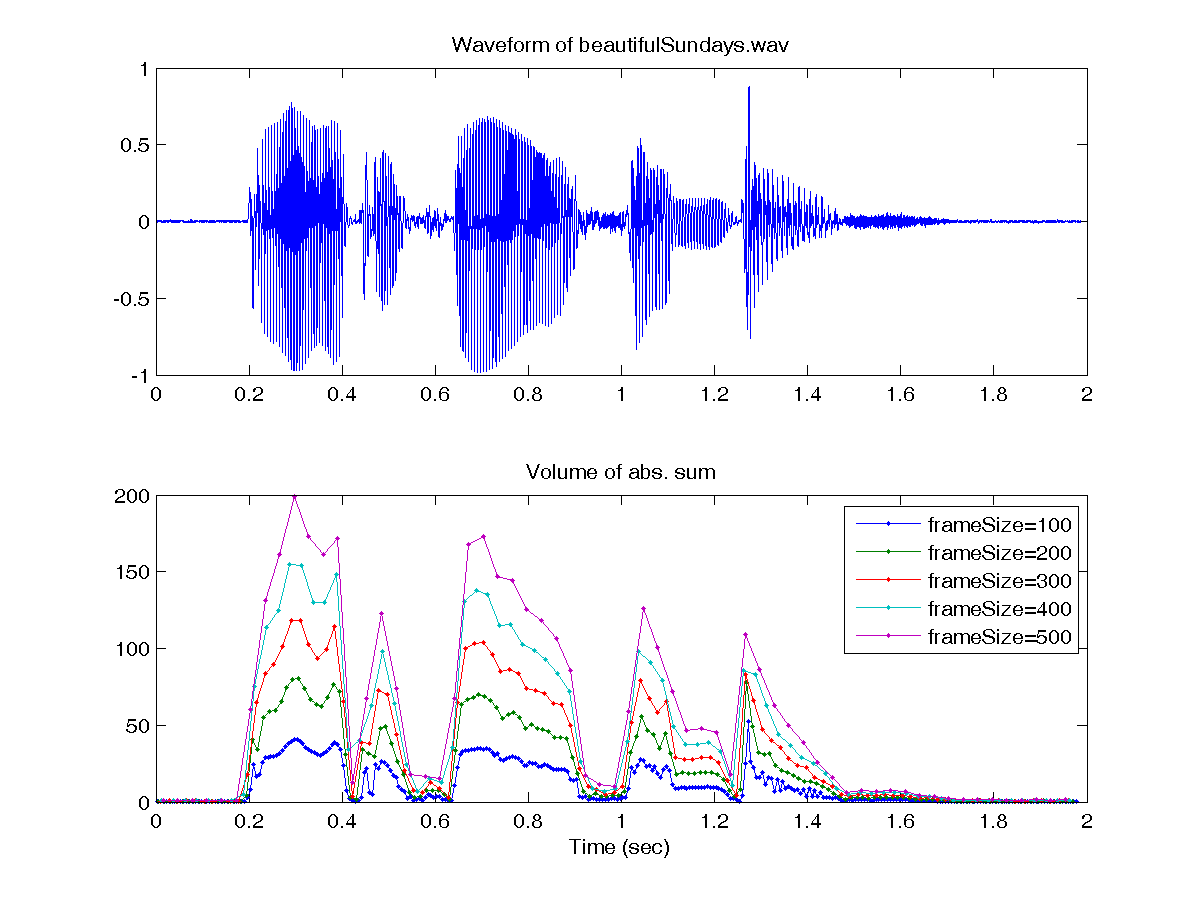2. Write an m-file script to repeat the previous sub-problem, but compute the volumes in terms of decibels. Your plot should look like this: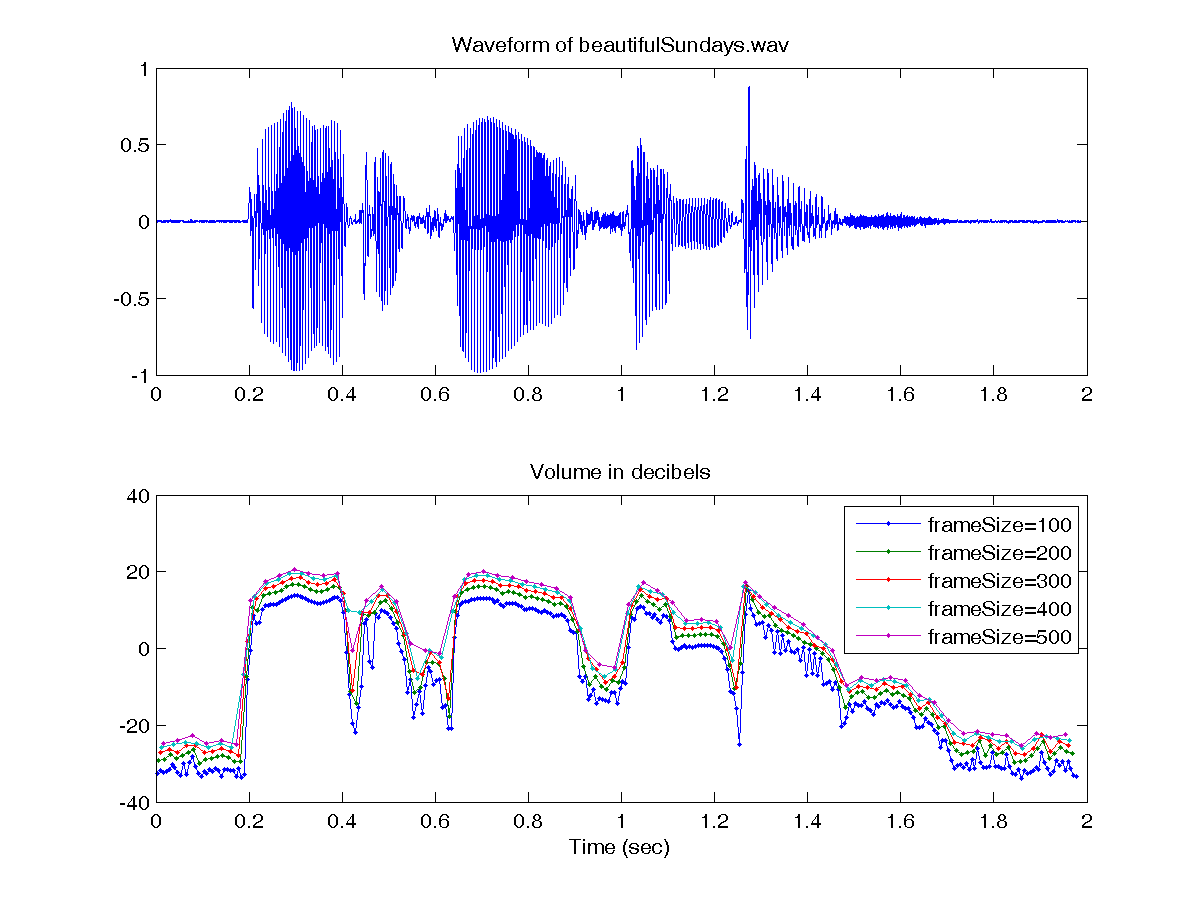Hint
• You can do the recording with either MATLAB or CoolEdit. But CoolEdit may be easier for you to edit the recordings, e.g., cut off leading or trailing slience, etc.
• Since each volume vector corresponds to a different time vector, you can put all volume vectors in a volume matrix, and all time vectors in a time matrix, and plot 5 volume curves all at once. The length of these 5 curves are not the same, hence you need to pad the time/volume matrix with NaN.
• Here are some functions in the SAP Toolbox that can make your job easier: frame2volume.m, frame2sampleIndex.m.

28. (***) Impact of the values of K on KCR: We can extend the definition of ZCR to KCR (k-crossing rate) which is the number of crossings over y = k in a frame. Write an m-file script that read your recording of "beautiful sundays" (as integers) and plot 7 KCR curves with K equal to 0, 100, 200, ..., 600. The frame size is 256 and the overlap is 0. You program should plot the waveform as well as the KCR curves on the same time axis. The plot should look like this: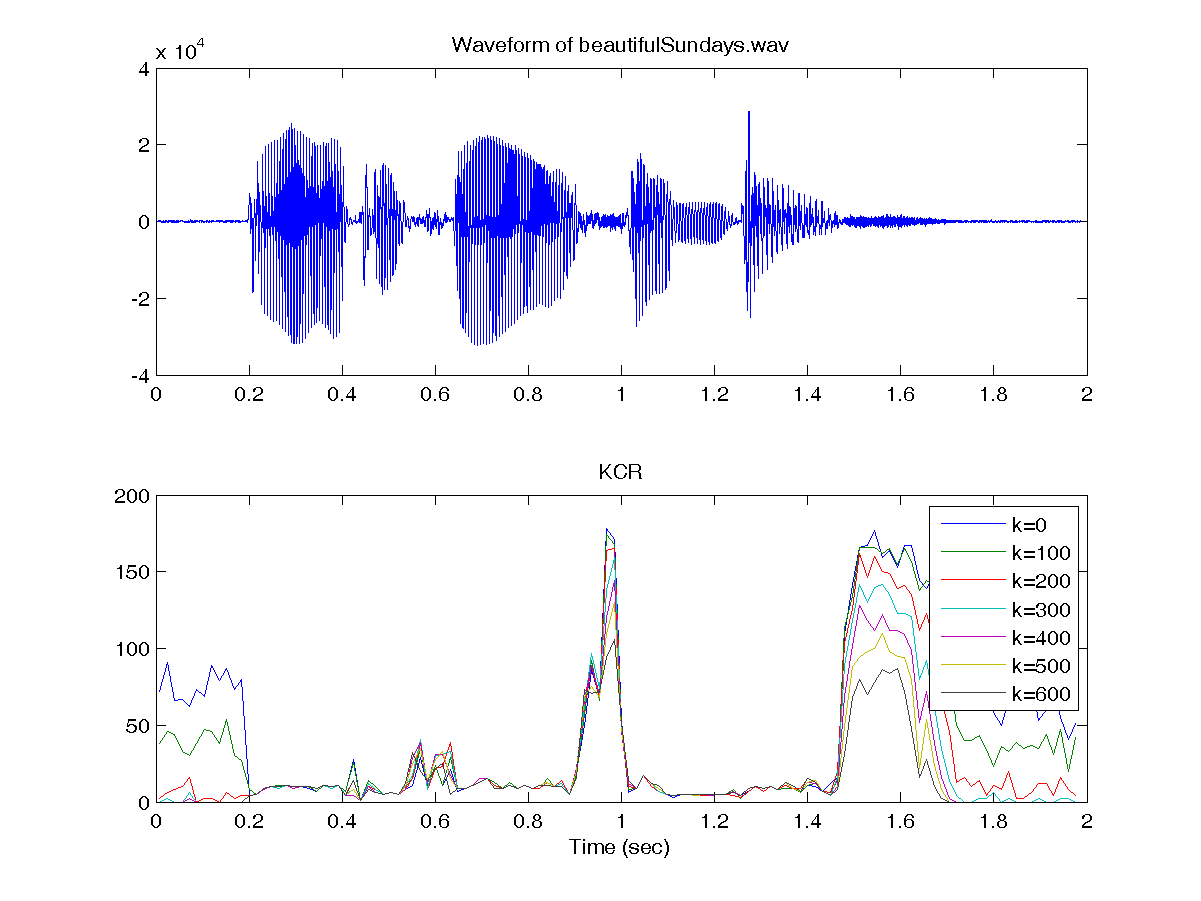Do these KCR curves have different but consistent behavior on voiced and unvoiced sounds? Can you use KCR for unvoiced/voiced detection?

Hint
You should use the function frame2zcr.m of the SAP Toolbox directly.

29. (***) Playback of music notes: This problem concerns the playback of music notes using the concatenation of sinusoids.
1. Write a m-file function which can take a sequence of music notes and return the audio signals for playback. The format is

wave=note2wave01(pitch, duration, fs)

where "pitch" is the vector of note pitch in semitones (with 0 indicating silence), "duration" is the vector of note duration in seconds, "fs" is the sample rate of the output wave signals for playback. Using the following script to try your function and see if you can identify the song. (my result for your reference) pitch= [55 55 55 55 57 55 0 57 60 0 64 0 62 62 62 62 60 64 60 0 57 55 55]; duration=[23 23 23 23 23 35 9 23 69 18 69 18 23 23 23 12 12 23 35 9 12 12 127]/64; fs=16000; wave=note2wave01(pitch, duration, fs); sound(wave, fs);
2. If you concatenate the sinusoids of two different notes directly, you can hear obvious noise due to the discontinuity between waveforms of these two notes. Can you find a method to eliminate the noise so it is more pleasant to hear it? (Hint: There are several methods to solve the problem.)
30. 請問判斷女聲或男聲，通常女聲音調會較高，男則低，可是若女聲跟男聲音調跟音量差不多時，還是可以稍微判斷出說話的人是男生還是女生，所以是否可以從音色上判斷男女，可以的話是如何判斷的?
31. 透過剔除聲音低於聽覺可以聽到的頻段，可壓縮聲音檔的大小，請問此種壓縮技術為何?
1. 霍夫曼編碼
2. 遮蔽效應
3. 非線性反應
4. 最小聽覺門檻

Audio Signal Processing and Recognition (音訊處理與辨識)